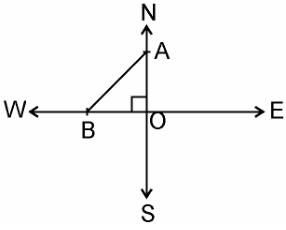# An aeroplane leaves an airport and flies due north at a speed of 1,000 km per hour. At the same time, another aeroplane leaves the same airport and flies due west at a speed of 1,200 km per hour. How far apart will be the two planes after 1(1/2) hours?

Distance covered by place left towards north = 1000 * 1.1/2

Distance covered by place left towards west = 1200 * 1.1/2 = 1800kmOn applying Pythagoras theorem to ΔAOB, we get

AB2 =AO2 + OB2

⇒ AB2 =(1500)2 + (1800)2

⇒ AB = √(2250000 + 3240000)

= √5490000

⇒ AB = 300√61 km

Hence, the distance between two aeroplanes will be 300√61 km.(1)(0)